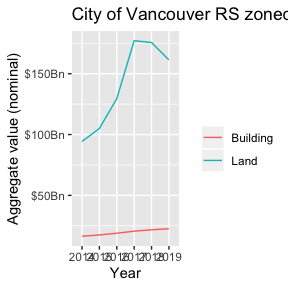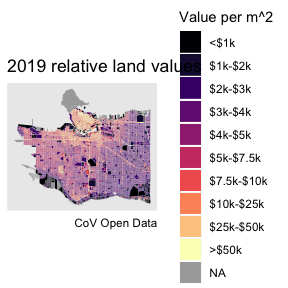# Demo

#### 2019-11-04

library(VancouvR)
library(dplyr)
#>
#> Attaching package: 'dplyr'
#> The following objects are masked from 'package:stats':
#>
#>     filter, lag
#> The following objects are masked from 'package:base':
#>
#>     intersect, setdiff, setequal, union
library(tidyr)
library(ggplot2)

### Get metadata for tax report

get_cov_metadata("property-tax-report") %>%
tail(10)
#> # A tibble: 10 x 4
#>    name           type   label         description
#>    <chr>          <chr>  <chr>         <chr>
#>  1 current_land_… int    CURRENT_LAND… The market value of the fee simple …
#>  2 current_impro… int    CURRENT_IMPR… The market value of the fee simple …
#>  3 tax_assessmen… text   TAX_ASSESSME… Year in effect for Current_Land_Val…
#>  4 previous_impr… int    PREVIOUS_IMP… This value is for the previous asse…
#>  5 previous_land… int    PREVIOUS_LAN… This value is for the previous asse…
#>  6 year_built     text   YEAR_BUILT    Year that the property was built. A…
#>  7 big_improveme… text   BIG_IMPROVEM… Year of major improvement to the pr…
#>  8 tax_levy       double TAX_LEVY      This is the total taxes printed on …
#>  9 neighbourhood… text   NEIGHBOURHOO… This is a 3-digit number assigned b…
#> 10 report_year    text   REPORT_YEAR   Report year

### Get an overview of land values in RS zones

aggregate_cov_data("property-tax-report",
group_by="tax_assessment_year as Year",
where="zone_name like 'RS-'",
select="sum(current_land_value) as Land, sum(current_improvement_value) as Building") %>%
mutate(Date=as.Date(paste0(as.integer(Year)-1,"-07-01"))) %>%
pivot_longer(c("Land","Building")) %>%
ggplot(aes(x=Year,y=value,color=name,group=name)) +
geom_line() +
scale_y_continuous(labels=function(x)paste0("$",x/1000000000,"Bn")) + labs(title="City of Vancouver RS zoned land values",color="",y="Aggregate value (nominal)") #> Downloading data from CoV Open Data portal### Get data for property tax report and property polygons tax_data <- get_cov_data(dataset_id = "property-tax-report", where="tax_assessment_year=2019", select = "current_land_value, land_coordinate as tax_coord") #> Downloading data from CoV Open Data portal #> Warning: NAs introduced by coercion to integer range property_polygons <- get_cov_data(dataset_id="property-parcel-polygons",format = "geojson") #> Downloading data from CoV Open Data portal ### Compute and plot relative land values plot_data <- property_polygons %>% left_join(tax_data %>% group_by(tax_coord) %>% summarize(current_land_value=sum(current_land_value)),by="tax_coord") %>% mutate(rlv=current_land_value/as.numeric(sf::st_area(geometry))) %>% mutate(rlvd=cut(rlv,breaks=c(-Inf,1000,2000,3000,4000,5000,7500,10000,25000,50000,Inf), labels=c("<$1k","$1k-$2k","$2k-$3k","$3k-$4k","$4k-$5k","$5k-$7.5k","$7.5k-$10k","$10k-$25k","$25k-$50k",">\$50k"),
ordered_result = TRUE))
#> Linking to GEOS 3.7.2, GDAL 2.4.2, PROJ 5.2.0
ggplot(plot_data) +
geom_sf(aes(fill=rlvd),color=NA) +
scale_fill_viridis_d(option="magma",na.value="darkgrey") +
labs(title="2019 relative land values",fill="Value per m^2",caption="CoV Open Data") +
coord_sf(datum=NA)